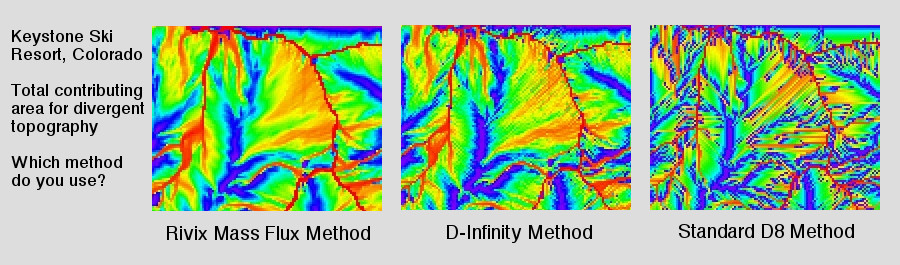### The RiverTools Mass Flux Method

On a hillslope flowlines may first diverge (spread out) before converging into channels at the bottom of the hill. RiverTools provides routines for analyzing both convergent and divergent flow. For convergent flow and the identification of individual channels, channel networks and basin boundaries, the well-known D8 algorithm is used. This algorithm tracks flow from each pixel to one of its eight neighbor pixels. The RiverTools implementation of the D8 algorithm is efficient and powerful, and can assign reasonable flow directions even in very complex and ambiguous situations without user intervention. However, the D8 algorithm is based on two simplifying assumptions that do not capture the geometry of divergent flow over hillslopes. These simplifications are: (1) the use of eight discrete flow angles, and (2) all flow leaving any given pixel (or the area contributing flow to the pixel) is assumed to flow into a single downstream neighbor pixel. While these simplifications are valid in regions where the flow is convergent, it is possible to do better on hillslopes where the flow is divergent by using an algorithm that allows (1) continuous flow angles, and (2) flow partitioning to two or more neighbor pixels.

The D-Infinity algorithms proposed by Tarboton (1997) provide a fairly robust and pragmatic solution to this problem, but they do not solve it in a rigorous manner. That is, the rule for partioning mass between two neighbor pixels is somewhat arbitrary and not based on mass balance. These algorithms strike a compromise between the limitations of D8 flow and other problems that occur when flow to more than two neighbor pixels is permitted. They are available within RiverTools via the Extract → D-Infinity Grid menu, and can be used to create a continuous angle flow grid, a slope grid, and a contributing area grid.

In RiverTools 3.0, a new method for partioning flow between neighbor pixels was introduced, called simply the Mass Flux Method, based on ideas from computational fluid dynamics. It has some basic elements in common with the DEMON algorithm that was proposed by Costa-Cabral and Burges (1994). This method treats each pixel as a control volume and uses a rigorous mass balance that

1. divides each pixel into four quarter-pixels,
2. computes a continuous flow angle for each, and then
3. computes total contributing area (TCA) and specific contributing area (SCA) using the actual fraction of flow that would pass through each of a pixel's four edges under a uniform rainrate.
The accuracy of this contributing area does not depend on whether rainrate is actually uniform or not, it is just a conceptual construct that allows the problem to be reformulated as a fluid dynamics (mass flux) problem. In this case, the total contributing area (TCA) is equivalent to a volumetric discharge of water, Q, divided by a constant rainrate, R, i.e. A = (Q/R). As with the D-Infinity method, flow from a given pixel (grid cell) will typically be partitioned between two neighbor pixels, except in the case of pixels that are single-pixel peaks or that lie on drainage divides. However, with the mass flux method, flow will only be to nearest neighbors, as opposed to one nearest and one diagonal neighbor as is the case with the D-infinity method. Any multiple-flow direction algorithm that sends flow to more than two downhill neighbors (except on peaks and ridges) is incorrect and subject to a problem called "overdispersion".

Note that we are speaking in terms of flows and mass balance even though our objective is to compute contributing areas on divergent surfaces as accurately as possible. The new Mass Flux algorithms are available in RiverTools via the Extract → Mass Flux Grid menu. In order to handle peaks and linear ridges as accurately as possible, grids based on quarter-pixels are created and saved (with QP for quarter-pixel in their name, along with their own RTI files) as an intermediate step. These have twice as many columns and rows as the original DEM, but are then integrated to create flow angle and area grids that have the same dimensions as the DEM. Continuous angle grids from the D-Infinity and Mass Flux methods look quite similar, but there can be significant differences between the area grids computed by the two methods as seen in the figure at the top of this page. Both the D-Infinity and Mass Flux algorithms use a D8 flow grid to resolve ambiguous flow situations in flats and pits.

You can experiment with these different algorithms by using DEMs in the Test_Surfaces folder in the data folder within the main RiverTools_4.0 application folder. While they can give significantly different results on a divergent surface, they all give similar values for the contributing areas of larger, channelized watersheds.

More information on the D8 and D-Infinity algorithms can be found here.